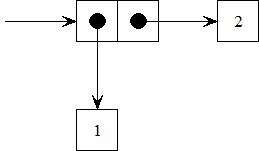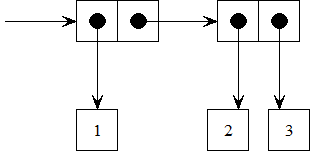## Thursday, September 30, 2010

### SICP 2.2: Line segments in a plane

From SICP section 2.1.2 Abstraction Barriers

Exercise 2.2 asks us to define a constructor and selectors for creating line segments in a plane. A line segment is represented by a starting point and an ending point. We'll also need to create a constructor and selectors for creating points in a plane, where a point is represented by an x and a y value. Finally, using these constructors and selectors we need to define a midpoint-segment procedure that takes a line segment as its argument and returns the midpoint of that segment. A procedure for printing points is provided.
(define (print-point p)   (newline)   (display "(")   (display (x-point p))   (display ",")   (display (y-point p))   (display ")"))
If you watched lecture 2B on compound data, you already know that the procedures for defining points in a plane are going to be very similar to the original procedures for defining rational numbers. All we need to do to construct a point is glue its two parts together.
; point constructor(define (make-point x y) (cons x y)); point selectors(define (x-point p) (car p))(define (y-point p) (cdr p))

The procedures for defining segments are almost the same, only the names will change.
; segment constructor(define (make-segment a b) (cons a b)); segment selectors(define (start-segment s) (car s))(define (end-segment s) (cdr s))

Remember that we're just gluing two "things" together. It only matters conceptually (which is pretty important, really) that the two things in this case are points, while before they were primitive numbers. The mechanism for combining them is the same.

Next we need to use the midpoint formula to define a procedure for computing the midpoint of a segment. The midpoint is defined as the average of the x-values and the y-values of the two endpoints of the segment.Our new procedure needs to take a segment and return a point.
(define (midpoint-segment s)    (make-point (/ (+ (x-point (start-segment s))                     (x-point (end-segment s)))                  2)               (/ (+ (y-point (start-segment s))                    (y-point (end-segment s)))                  2)))

There are a few examples illustrating the midpoint formula on Purplemath. I'll use those to test with.

Find the midpoint between (–1, 2) and (3, –6).
Answer: P = (1, -2)
> (define a (make-point -1 2))> (define b (make-point 3 -6))> (define s (make-segment a b))> (define m (midpoint-segment s))> (print-point m)(1,-2)

Find the midpoint between (6.4, 3) and (–10.7, 4).
Answer: P = (–2.15, 3.5)
> (define a (make-point 6.4 3))> (define b (make-point -10.7 4))> (define s (make-segment a b))> (define m (midpoint-segment s))> (print-point m)(-2.1499999999999995,7/2)

Related:

For links to all of the SICP lecture notes and exercises that I've done so far, see The SICP Challenge.

## Thursday, September 23, 2010

### SICP 2.1: Rational numbers

From SICP section 2.1.1 Example: Arithmetic Operations for Rational Numbers

Exercise 2.1 asks us to define a better version of make-rat that handles both positive and negative arguments. Here's the last version from the text, along with several example cases to show how it works:
(define (make-rat n d)  (let ((g (gcd n d)))    (cons (/ n g) (/ d g))))> (define x (make-rat 1 2))> (print-rat x)1/2> (define x (make-rat -1 -2))> (print-rat x)-1/-2> (define x (make-rat 1 -2))> (print-rat x)1/-2> (define x (make-rat -1 2))> (print-rat x)-1/2

The new version of make-rat should normalize the sign so that if the resulting rational number is positive, both the numerator and denominator are positive. If the rational number is negative, only the numerator should be negative.

We can correct make-rat by checking to see if the denominator is negative. If it is, we need to multiply both the numerator and denominator by -1 to normalize the result. If not, we just pass the arguments bare to cons.
(define (make-rat n d)  (let ((g (gcd n d)))    (if (< d 0)        (cons (/ (* n -1) g) (/ (* d -1) g))        (cons (/ n g) (/ d g)))))

We can use the same definitions as before to see the results from the new make-rat procedure.
> (define x (make-rat 1 2))> (print-rat x)1/2> (define x (make-rat -1 -2))> (print-rat x)1/2> (define x (make-rat 1 -2))> (print-rat x)-1/2> (define x (make-rat -1 2))> (print-rat x)-1/2

Related:

For links to all of the SICP lecture notes and exercises that I've done so far, see The SICP Challenge.

## Wednesday, September 22, 2010

### SICP Lecture 2B: Compound Data

Structure and Interpretation of Computer Programs
Compound Data
Covers Text Section 2.1: Introduction to Data Abstraction

You can download the video lecture or stream it on MIT's OpenCourseWare site. It's also available on YouTube.

This lecture is presented by Professor Harold Abelson

Chapter 1 of SICP was all about procedures using primitive data, and how to build higher and higher abstractions using procedures. The next chapter will be all about combining primitive data into compound data abstractions.

The fundamental means of combining primitive data in Scheme is to glue two things together into a pair. We use the cons function to combine two things together to make a pair. Once you've combined them you need something to get the two separate parts back. To do that you use the car and cdr functions.

(cons x y) - constructs a pair whose first part is x and second part is y.

(car p) - selects the first part of the pair p.

(cdr p) - selects the second part of the pair p.

The convention for drawing diagrams of pairs is called box-and-pointer notation.The formal way of describing the behavior of cons, car, and cdr, and how they interact with one another is called the axiom for pairs:

For any x and y:
(car (cons x y)) is x
(cdr (cons x y)) is y

Representing rational numbers

The text defines a system for representing rational numbers using pairs.
(define (make-rat n d) (cons n d))(define (numer x) (car x))(define (denom x) (cdr x))

These functions are called a constructor (make-rat) and selectors (numer and denom). Once we have the simple data abstraction in place, we can use the abstraction to define other procedures that work with rational numbers using the rules of rational number arithmetic.
(define (add-rat x y)   (make-rat (+ (* (numer x) (denom y))                (* (numer y) (denom x)))             (* (denom x) (denom y))))(define (sub-rat x y)   (make-rat (- (* (numer x) (denom y))                (* (numer y) (denom x)))             (* (denom x) (denom y))))(define (mul-rat x y)   (make-rat (* (numer x) (numer y))             (* (denom x) (denom y))))(define (div-rat x y)   (make-rat (* (numer x) (denom y))             (* (denom x) (numer y))))(define (print-rat x)   (newline)   (display (numer x))   (display "/")   (display (denom x)))

Data abstraction separates the use of a data object from its representation. You could define add-rat and the other procedures without using data abstraction by directly using cons, car, and cdr. The point of isolating the use from the representation is that you have to capture the idea of a conceptual entity.

Compound Data

Like procedure definition, the real power of compound data is that we can use it to create building blocks that can themselves be used to create more complex data structures. Using the same method we used for creating rational numbers, we could also create a set of functions for representing points in a plane. From those points we can build line segments, from line segments we can build two-dimensional shapes. We could keep going and build as many abstractions on top of each other as we need to represent any system we want.

Closure
- the means of combination in the system are such that when you put things together with them you can then put those things together using the same means. The system is closed. (This use of the word "closure" is in the same sense as it's used in abstract algebra. The Lisp community also uses the same word for an unrelated concept.)

Once you can combine two things, the closure property allows you to combine as many things as you want.We'll see later in the text that this is how lists are formed in Scheme.

What are pairs really?

The last section of the lecture shows that pairs can be build out of nothing at all. If Scheme didn't have primitive functions cons, car, and cdr we could define them ourselves.
(define (cons a b)  (lambda (pick)     (cond ((= pick 1) a)           ((= pick 2) b))))(define (car x) (x 1))(define (cdr x) (x 2))

In this implementation cons returns a procedure, not a pair. The definitions for car and cdr take the procedure supplied by cons and apply it to 1 and 2 respectively. If this system satisfies the axiom for pairs that we saw earlier, then it's a perfectly reasonable implementation. In the lecture, mechanical substitution is used to prove that this system does satisfy the axiom for pairs.

Related:

For links to all of the SICP lecture notes and exercises that I've done so far, see The SICP Challenge.